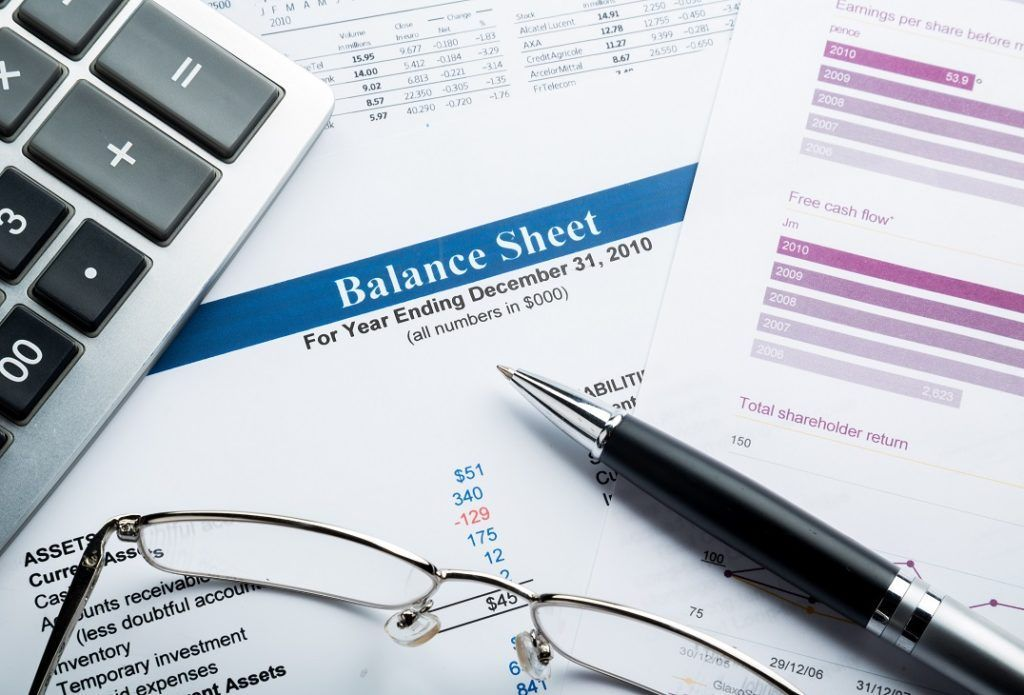# Net Book Value

How accountants record a company's assets

## What is Net Book Value?

Net book value (NBV) refers to a company’s assets or how the assets are recorded by the accountant. NBV is calculated using the asset’s original cost – how much it cost to acquire the asset – with the depreciation, depletion, or amortization of the asset being subtracted from the asset’s original cost.### Subtracting Depreciation/Depletion/Amortization

Over time, assets lose some of their value. When calculating NBV, the depletion or depreciation and any amortization of the asset’s value must be subtracted from the original cost over the course of the asset’s useful life. (Every asset has a reasonable period of time over which it can be used or useful.)

Salvage value is another factor to be considered. Some assets may have more value that can be derived from them after the end of their useful life. For example, consider a logging company that purchases a hauling truck. It may have a salvage value that will make it useful in another way instead of simply scrapping the truck once it no longer functions in its hauling capacity.

What all of the above means is that the NBV of an asset should decrease fairly steadily and predictably over the useful life of the asset. When it reaches the end of its useful life, the NBV should be equal to its salvage value.

### Calculating Net Book Value

The formula for calculating NBV is as follows:

Net Book Value = Original Asset Cost – Accumulated Depreciation

Where:

• Accumulated Depreciation = Per Year Depreciation x Total Number of Years

### Sample Calculation of Net Book Value

Let’s put in the example of the logging truck mentioned above. If the logging company purchased the truck for \$200,000 and the truck depreciated \$15,000 per year for 4 years, the calculation of NBV would look like below:

Accumulated Depreciation = \$15,000 x 4 years = \$60,000

Net Book Value = \$200,000 – \$60,000 = \$140,000

In our example, the NBV of the logging company’s truck after four years would be \$140,000.

### Importance of Net Book Value

Net book value is among the most popular financial metrics around. It is especially true when used to help give value to a company – either for the company’s own accounting records, if the company is considering liquidation, or if another company is considering taking over the business.

Market value is another important metric; however, NBV and market value typically aren’t equal. Market value changes every time prices change.

It’s also important to understand that NBV is affected by how rapidly depreciation is reported and calculated by a company. Depreciation is always accumulated, however, some companies choose to accelerate depreciation.

In such a case, the NBV will be significantly lower than the market value for the first few years of the asset’s useful life. NBV is incredibly important for a company to know. It makes for fairer and more accurate accounting records and helps to express a true approximation of the company’s total value.

We hope you’ve enjoyed reading CFI’s explanation of Net Book Value. CFI is the official provider of the Financial Modeling and Valuation Analyst (FMVA)™ certification program, designed to transform anyone into a world-class financial analyst.

To keep learning and developing your knowledge of financial analysis, we highly recommend the additional CFI resources listed below:

• Depreciation Methods